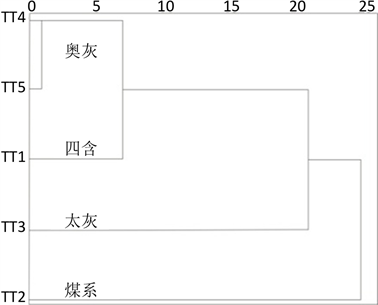﻿ 基于多元矩阵模型的童亭煤矿突水水源识别

# 基于多元矩阵模型的童亭煤矿突水水源识别Source Identification of Water Bursting in Tongting Coal Mine Based on Multivariate Matrix Model

Abstract: Even in the context of the country’s energy structure adjustment, coal is still one of the major energy sources in China for a certain period of time. The task of coal mine safety production is still arduous. As one of the five disasters of coal mine, water disaster seriously threatens the production safety of coal mine. In order to reduce the occurrence of water disaster accidents in coal mines, the most important thing is to identify the source of water inrush effectively. Based on 7 kinds of conventional hydro-chemical indexes of Na+ + K+, Mg2+
, Ca2+, Cl−, SO2-4 , HCO-3 , and TDS from four aquifers of Tongting coal mine, a multivariate matrix model for identification of water inrush in mine is established and the possibility of the water inrush samples belonging to each aquifer can be determined by the combination of ions. Taking the combination of Na+ + K+, Mg2+ and Ca2+ as an example, the possibility of water inrush belonging to fourth aquifer, coal bearing sandstone aquifer, Taiyuan formation limestone aquifer and Ordovician limestone is −0.2978, −0.0396, 0.1101, 0.8486 respectively. Therefore, the ion combination shows that the source of water inrush samples is Ordovician limestone water. Through the calculation results of other groups of ions, the source of water inrush can be judged similarly. The results show that there are 0 groups of water inrush from coal bearing sandstone, 2 groups from Taiyuan formation limestone aquifer, 4 groups from the fourth aquifer, 29 groups from Ordovician limestone aquifer, and the average value of each group is also shown as Ordovician limestone aquifer. Accordingly, it can be considered that the source of water inrush is Ordovician limestone aquifer water. Results show that the principle is simple, and less limited by water samples and the index number, and has good recognition effect, and water recognition rate can reach 82.86% in Tongting coal mine, which can provide a reference for prevention and control of water disasters in coal mine.

1. 引言

2. 矿井水文地质特征

3. 研究方法

$CF=P$$C=\left[\begin{array}{cccc}{C}_{11}& {C}_{12}& \cdots & {C}_{1n}\\ {C}_{21}& {C}_{22}& \cdots & {C}_{2n}\\ ⋮& ⋮& \ddots & ⋮\\ {C}_{n-1,1}& {C}_{n-1,2}& \cdots & {C}_{n-1,n}\\ 1& 1& 1& 1\end{array}\right]$$F=\left[\begin{array}{c}{F}_{1}\\ {F}_{2}\\ ⋮\\ {F}_{n-1}\\ {F}_{n}\end{array}\right]$$P=\left[\begin{array}{c}{P}_{1}\\ {P}_{2}\\ ⋮\\ {P}_{n-1}\\ {P}_{n}\end{array}\right]$ (1)

4. 模型的构建及应用Table 1. Routine hydrochemical indexes of Tongting mine (mg/L)

$\left[\begin{array}{cccc}{C}_{11}& {C}_{12}& {C}_{13}& {C}_{14}\\ {C}_{21}& {C}_{22}& {C}_{23}& {C}_{24}\\ {C}_{31}& {C}_{32}& {C}_{33}& {C}_{34}\\ 1& 1& 1& 1\end{array}\right]\left[\begin{array}{c}{F}_{1}\\ {F}_{2}\\ {F}_{3}\\ {F}_{4}\end{array}\right]=\left[\begin{array}{c}{P}_{1}\\ {P}_{2}\\ {P}_{3}\\ 1\end{array}\right]$ (2)

$\left[\begin{array}{cccc}{\rho }_{1}\left({\text{Na}}^{+}+{\text{K}}^{+}\right)& {\rho }_{2}\left({\text{Na}}^{+}+{\text{K}}^{+}\right)& {\rho }_{3}\left({\text{Na}}^{+}+{\text{K}}^{+}\right)& {\rho }_{4}\left({\text{Na}}^{+}+{\text{K}}^{+}\right)\\ {\rho }_{1}\left({\text{Mg}}^{2+}\right)& {\rho }_{2}\left({\text{Mg}}^{2+}\right)& {\rho }_{3}\left({\text{Mg}}^{2+}\right)& {\rho }_{4}\left({\text{Mg}}^{2+}\right)\\ {\rho }_{1}\left({\text{Ca}}^{2+}\right)& {\rho }_{2}\left({\text{Na}}^{+}+{\text{K}}^{+}\right)& {\rho }_{3}\left({\text{Na}}^{+}+{\text{K}}^{+}\right)& {\rho }_{4}\left({\text{Na}}^{+}+{\text{K}}^{+}\right)\\ 1& 1& 1& 1\end{array}\right]\left[\begin{array}{c}{F}_{1}\\ {F}_{2}\\ {F}_{3}\\ {F}_{4}\end{array}\right]=\left[\begin{array}{c}{P}_{1}\\ {P}_{2}\\ {P}_{3}\\ 1\end{array}\right]$ (3)Table 2. Influence of aquifer on water bursting water sample and water source discrimination in Tongting coal mineFigure 1. R cluster analysis of Tongting mine

5. 结论

 温廷新, 张波, 邵良杉. 矿井突水水源识别预测研究——以新庄孜矿为例[J]. 中国安全科学学报, 2014, 24(2): 100-106.

 Sun, L.H. and Gui, H.R. (2012) Establishment of Water Source Discrimination Model in Coal Mine by Using Hydrogeochemistry and Statistical Analysis: A Case Study from Renlou Coal Mine in Northern Anhui Province, China. Journal of Coal Science and Engineering (China), 18, 385-389.
https://doi.org/10.1007/s12404-012-0409-0

 Sun, L., Chen, S. and Gui, H. (2016) Source Identification of Inrush Water Based on Groundwater Hydrochemistry and Statistical Analysis. Water Practice & Technology, 11, 448-458.
https://doi.org/10.2166/wpt.2016.049

 桂和荣. 矿区地下水水文地球化学演化与识别[M]. 北京: 地质出版社, 2007.

 陈松. 基于三维地质结构模型的宿南矿区地下水演化与识别[D]: [博士学位论文]. 徐州: 中国矿业大学, 2014.

 张俊, 李余生, 林曼利, 等. 淮南张集矿水文地球化学特征及水源识别[J]. 水文地质工程地质, 2014, 41(6): 32-37.

 李法胜, 于政中. 矩阵模型在最优树种组成研究中的应用[J]. 北京林业大学学报, 1992, 14(2): 23-30.

 蔡笃程, 程立生. 二斑叶螨实验种群的矩阵模型及其应用[J]. 热带农业科学, 1997(3): 28-32.

 胡华进, 徐治皋. 电站热力系统循环组合法及其矩阵模型[J]. 中国电机工程学报, 1998, 18(1): 12-15.

 刘剑民, 王继仁, 何治良. 基于多元混合模型的煤矿矿井突水水源分析[J]. 中国安全科学学报, 2013, 23(12): 95-100.

 孙希奎, 施现院, 梁丽鹏, 等. 矿井涌水水源分析的多元矩阵模型及应用[J]. 煤炭技术, 2016, 35(12): 167-169.

 谢中华, 何健. 童亭井田10煤层底板灰岩水的水文地质条件分析[J]. 淮南职业技术学院学报, 2002, 2(3): 58-61.

 刘鑫. 临涣矿区主要突水含水层的水文地球化学研究[D]: [硕士学位论文]. 合肥: 合肥工业大学, 2013.

Top